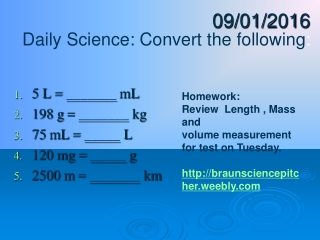Download Presentation09/01/2016

# 09/01/2016

Télécharger la présentation## 09/01/2016

- - - - - - - - - - - - - - - - - - - - - - - - - - - E N D - - - - - - - - - - - - - - - - - - - - - - - - - - -
##### Presentation Transcript

1. 09/01/2016 Daily Science: Convert the following: • 5 L = _______ mL • 198 g = _______ kg • 75 mL = _____ L • 120 mg = _____ g • 2500 m = _______ km Homework: Review Length , Mass and volume measurement for test on Tuesday. http://braunsciencepitcher.weebly.com

2. Volume A measurement of the amount of space something takes up.

3. From atoms… to planets If it exists in nature, it takes up space…

4. Measuring Volume A. Liquids B. Solids a. Rectangular b. Irregular

5. A. Measuring the volume of liquids with a Graduated Cylinder • Place the graduated cylinder on a flat surface.

6. Reading the Graduated Cylinder • Your eye should be level with the top of the liquid You should read to the bottom of the MENISCUS or curve

12. What is the volume shown?

13. What is the volume shown? 37.5 mL

14. B. Measuring the volume of solids

15. a. Measuring Volume of Rectangular solids • measure with a ruler the length (L), width (W) and height (H) of the object. • multiply the 3 numbers together. • The product is the volume of the object. • unit of measure would be mm3, cm3, m3, etc.

16. Volume By Displacement • Fill the graduated cylinder at least halfway with water. • Record the volume of the water. • Drop the object into the water without splashing.

17. Record the volume of the water and the object together. • Subtract the volume of the water from the volume of the water and object. This is the volume of the object. • Remember- Convert liquid volume units (ml) to solid volume units (cm3)

18. Today’s Directions • Find the volume of the rectangle by measuring the length, width, and height with a ruler and then multiplying. • Find the volume of the rectangle by using the displacement method. • Find the volume of the glass bead by using the displacement method. • Don’t forget the correct units of measure.

19. Measuring Volume By Displacement